Saturday, March 25, 2023
HomePower ElectronicsSlew Rate for OP-AMP, Square and Sinusoidal Wave - How to Calculate...

# Slew Rate for OP-AMP, Square and Sinusoidal Wave – How to Calculate It

Slew Rate of an Op-Amp is a critical factor in determining the overall performance of the electronic circuit. This post will discuss in detail about what is Slew Rate (SR), how to calculate Slew Rate for OP-AMP, square and sinusoidal waveform and its applications, advantages as well as disadvantages.

## What is Slew Rate

The maximum possible rate of change of output voltage of Op-Amp with respect to time is referred to as Slew Rate. In other words, it can be defined as the parameter that describes the rate of variation of output voltage per specified unit time, when the inputs change rapidly.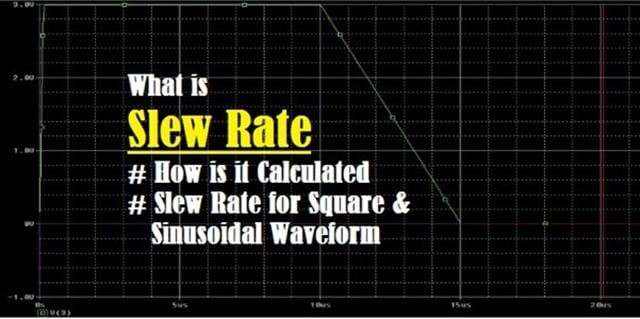Fig. 1 – Introduction to SR

The unit of Slew Rate is V/µsec (Volts/microsecond). For Example – If a device has a SR of 10 V/ µsec, it simply means that the voltage can be varied by 10 V in 1 μs. SR is typically measured by applying large signal step to the Op-Amp and the rate of change of output signals amplitude from 10 % to 90 % is measured. It is directly proportional to temperature i.e. as the temperature rises, SR decreases. Amplifiers with higher SR have higher current consumption.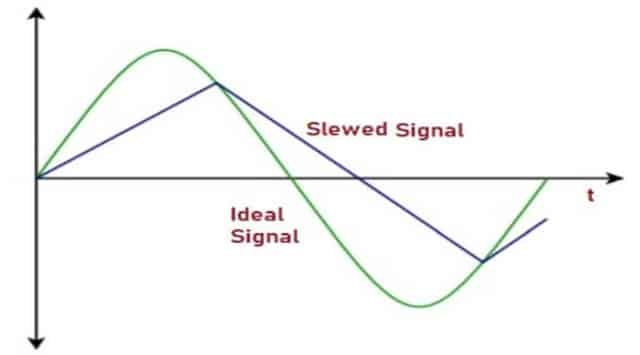Fig. 2 – Signal Distortion by Slewing

## How is Slew Rate Calculated

Slew Rate is calculated based on the rate of change of waveform of output signal called as Slope. Fig. 3 (a) shows the slope of the output signal and Fig. 3 (b) shows the transition from 10% to 90% and is called as “Rise Time”. SR can be calculated at any point on the waveform. This is defined by the equation: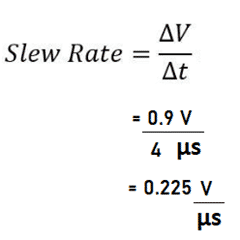Suppose the step response is ten times larger, for the same amount of rise time, then SR is:We can conclude that for the same rise time, Slew Rate can be 10 times larger due to the change in the Voltage.Fig. 3 – SR Calculation

## Slew Rate for Pulse Signals

If a pulse signal or square wave is applied to the input, the output is also expected to be a pulse wave but practically Slew Rate limits the rising and falling edges. The rising and falling edges causes oscillations in the Amplifier as shown in the Fig. 4.

To avoid this, the Op-Amp’s internal circuit contains a compensation capacitor i.e. a capacitor is connected inside of the op-amp IC, which improves the stability of the amplifier and prevents the oscillations across the output. This compensation limits the SR of the Operational Amplifier and since this is internal, it cannot be altered.Fig. 4 – Schematic Representation of Output Compensation

Some Op-Amps use compensation Capacitor externally, as a feedback loop to the Op-Amp and SR can be controlled to some extent.

## SR for Sinusoidal Waveform

Slew Rate limiting affects Sinusoidal Waveform. To know how fast a sinusoidal waveform changes, its necessary to find out the first derivative with respect to time.

Let us consider Sinusoidal waveform having input frequency ‘f’, amplitude ‘A’, varying with time ’t’.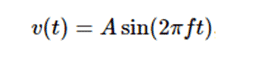Slew Rate is the slope or time derivative of waveform. When Cosine function reaches values ‘1’ and ‘-1’ as shown in the Fig. 4, SR is maximum. It is also at its highest, at zero crossings of a Sine wave i.e. t = 0. dv/ dt is the rate of change of signal. To find max dv/dt, substitute t = 0 in the below equation.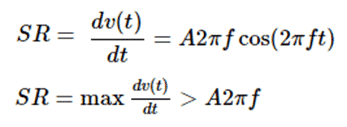We can infer that Slw Rate is directly proportional to frequency and amplitude of the waveform. Hence high amplitude, high frequency signals require op-amps with high SR. It is used to determine performance of the amplifier, usually by defining how fast a signal can slew at the output of the amplifier. Full Power Bandwidth (FPBW), also known as large signal bandwidth, is the highest frequency (fmax) that the amplifier can produce at its largest output voltage swing without Slewing Induced Distortion. FPBW or fmax can be found from rearranging the above equation: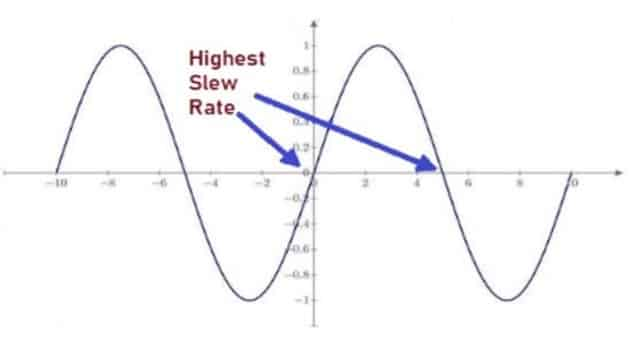Fig. 5 – SR for Sinusoidal Waveform

## Slew Rate of Ideal Op-Amp

The time delay should be negligible for an ideal Op-Amp. Irrespective of the changes of input voltage, the ideal Op-Amp must produce output voltage since time delay is negligible. According to the equation,From the above equations, we can say that the Slew Rate of ideal Op-Amp is infinite.

## Datasheet of Op-Amp

The Datasheet of an Operational Amplifier specifies SR for “Rising Signal” called as “Positive SlewRate” and “Falling Signal” called as “Negative SlewRate”. Datasheets of some devices might not differentiate between rising and falling signal as shown in the Fig. 6.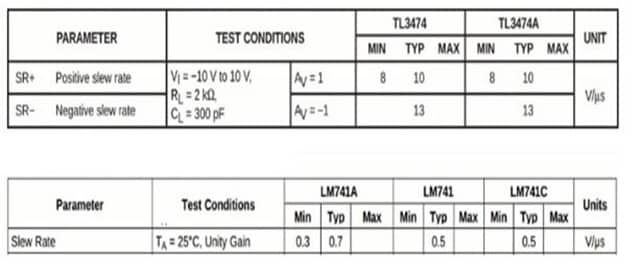Fig. 6 – Datasheet of Different Amplifiers

• Helps in determining input frequency and amplitude of the Amplifier.
• Prevents distortion of the output.
• Higher the Slew Rate, better is the reproduced signal.
• Very fast SR Op-amps require low impedance power supply.

• If the Slew Rate exceeds, the output waveform is distorted.
```Also Read:
Digital Communication - Introduction, Basic Components, How Signal Process Works
Sonar - Types, Architecture, How it works, Applications and Advantages```Laxmi Ashrit
Laxmi is a B.E (Electronics & Communication) and has work experience in RelQ Software as Test Engineer and HP as Technical support executive. She is an author, editor and partner at Electricalfundablog.
RELATED ARTICLES